﻿ 基于联合深度学习的地震数据随机噪声压制
 石油地球物理勘探2021, Vol. 56Issue (1): 9-25, 56  DOI: 10.13810/j.cnki.issn.1000-7210.2021.01.0020文章快速检索 高级检索

### 引用本文ZHANG Yan, LI Xinyue, WANG Bin, LI Jie, DONG Hongli. Random noise suppression of seismic data based on joint deep learning. Oil Geophysical Prospecting, 2021, 56(1): 9-25, 56. DOI: 10.13810/j.cnki.issn.1000-7210.2021.01.002.### 作者简介董宏丽, 黑龙江省大庆市高新技术开发区发展路199号东北石油大学人工智能能源研究院, 163318。Email:shiningdhl@vip.126.com

### 文章历史

1 东北石油大学计算机与信息技术学院, 黑龙江大庆 163318;
2 东北石油大学人工智能能源研究院, 黑龙江大庆 163318

Random noise suppression of seismic data based on joint deep learning
ZHANG Yan , LI Xinyue , WANG Bin , LI Jie , DONG Hongli1 Institute of Computer and Information Techno-logy, Northeast Petroleum University, Daqing, Heilongjiang 163318, China;
2 Artificial Intelligence Energy Research Institute, Northeast Petroleum University, Daqing, Heilongjiang 163318, China
Abstract: Random noise suppression is the key task of seismic data processing. To improve SNR (signal-to-noise ratio) and increase the efficiency and accuracy of following processing and interpretation, appropriate suppression methods should be used for noises induced by different mechanisms and with different characteristics. Applicable denoising methods based on deep learning usually focus on feature extraction in time or frequency domain, which result in over-smoothed or blurred textures in local zones. In addition, the kernel of a traditional convolution neural network is usually set to be a small and fixed block, which limits the size of the receptive field and reduces the diversity of the target characteristics extracted from seismic data. This paper proposes a method of random noise suppression based on joint deep learning. Firstly, features in both time domain and frequency domain are considered, and the joint error is used to define the loss function to improve effect of various extracted features. Secondly, by analyzing the influence of the kernel size and network depth on the size of the receptive field, the method of expanding convolution is used to extract more diverse features and reduce the loss of details of seismic data. Thirdly, according to the similarity between the input and output samples of the network, a residual learning strategy is introduced. Finally, the batch normalization (BN) algorithm is used to accelerate the convergence of the model and improve denoising efficiency. Compared with similar algorithms, the method proposed in this paper has a better effect on preserving the features of events and provides higher SNR.
Keywords: noise suppression    deep learning    joint loss function    expanded convolution    residual network    texture    signal-to-noise ratio (SNR)
0 引言

(1) 通常仅关注单一时域或者传统变换域(如傅里叶变换域、小波变换域等)的特征提取。如韩卫雪等提出的用于地震数据去噪的网络模型，与常规地震数据去噪算法相比，具有更好的去噪效果，但由于仅考虑时域提取特征，导致去噪过程中出现细节的丢失。Zhang等提出的基于残差学习的卷积去噪网络(Residual Learning of Deep CNN for Image Denoising，DnCNN)算法，采用卷积残差学习框架，从函数回归角度出发，用卷积神经网络(Convolutional Neural Networks，CNN)将噪声从含噪图像中分离出来。Yu等系统地介绍了DnCNN算法用于地震数据压制的过程并讨论了CNN的超参数设置问题。Wang等也将DnCNN模型应用于地震数据噪声压制，取得了较好的效果。Zhao等从图像块大小、卷积核大小、网络深度、训练集等方面对原始DnCNN进行了改进，使其适用于低频和沙漠区地震资料非高斯噪声的抑制。Dong等在此基础上，采用自适应DnCNN算法对沙漠地震资料进行去噪处理，有效提高了低频资料的信噪比。Yang等扩展了原有的DnCNN模型，构造了地震数据随机噪声衰减的卷积神经网络(CNN for Randon Noise Attenuation of Seismic Data, SDACNN)，将激活函数替换为指数线性单元(Exponential Linear Units，ELU)以增强网络的鲁棒性。以上方法均可以归结为基于DnCNN模型及其改进的去噪方法，而DnCNN仅关注时域的特征提取，忽略了数据在频域的特征，导致去噪地震数据中纹理特征损失较大。地震数据中同相轴的纹理特征是判断油气贮藏位置的关键，若考虑结合频域的特征，可在一定程度上抑制假频、使纹理保持效果得到较大的提升。Zhang等和Chen等基于无监督学习中自编码的概念，采用自编码的方式将地震数据降维以提取主要特征，再通过上采样层恢复到原尺寸，自适应地学习有效信号，实现无监督的地震数据随机噪声抑制，取得了较好的效果，但也忽略了地震数据在频域的特征，性能仍存在较大的提升空间。

Liu等提出的多级小波卷积神经网络(Multi-level Wavelet CNN，MWCNN)模型考虑图像的小波域分布特性，提出一种多级小波CNN框架，将离散小波变换与卷积网络结合，充分考虑了图像的频域特征。Zhu等利用短时傅里叶变换(Short-time Fourier Transform，STFT)将时域数据转化为频域数据，将实部和虚部传入卷积神经网络，网络根据输入数据产生信号和噪声掩膜，再利用相关掩膜进行估计，得到地震信号和噪声的频域系数，最后利用逆STFT得到时域去噪信号和噪声。以上两种方法的卷积处理过程仅在频域中进行，忽略了时域的特征，因此在地震数据能量较弱的区域，无法仅根据有限的特征判别真实数据和噪声数据，从而导致局部纹理模糊的现象。

(2) 使用的卷积核通常为较小的固定尺寸，提取地震数据的特征信息不充分，影响感受野大小。相同条件下，如果想得到相同范围的感受野就只能增加网络深度，但网络深度的增加会使提取的特征越来越高级，导致地震数据丢失较多的细节信息。例如Zhang等、韩卫雪等和其他部分学者基于DnCNN模型的改进[23-26]均使用固定大小为3×3的卷积核，学习到的噪声不充分，且具有较深的层数，容易造成过拟合现象。由于没有充分权衡卷积核大小与网络深度之间的关系，容易出现训练时间较长和细节信息丢失的问题。李传朋等的去噪卷积神经网络模型采用3×3与5×5的卷积核相结合的方法，去噪效果明显提高。

1 方法原理 1.1 网络模型结构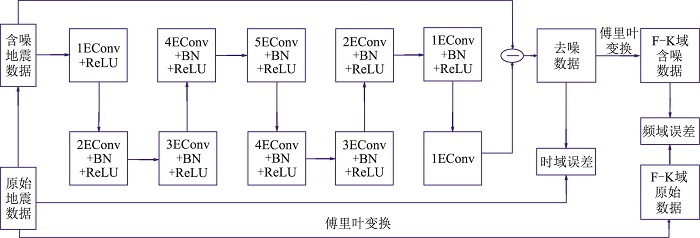图 1 网络模型结构图

(1) 联合时、频域的损失函数。由于当前大多数基于深度学习的去噪模型忽略时域与频域的联系，导致数据纹理细节损失较大。本文充分考虑时域和频域的特征，将傅里叶变换与卷积神经网络相结合，利用时域和频域联合误差定义损失函数，改善去噪效果，消除假频。

(2) 扩充卷积。为了平衡卷积核大小与网络深度之间的关系，采用扩充卷积的方式，在保留了传统3×3卷积优点的基础上，通过调整扩充因子增加感受野。从第1层至第10层，扩充因子分别设置为1、2、3、4、5、4、3、2、1、1，网络各层感受野大小分别为3、7、13、21、31、39、45、49、51、53。相同条件下，Dn-CNN模型如果达到大小为53的感受野，则需要26层的网络深度，但网络层数过多会导致丢失较多的细节信息。

(3) 残差网络学习。由于网络的输入与输出具有很强的相似性，残差学习更适合于地震数据的去噪。网络模型通过提取大量含噪数据样本学习噪声的特征，将含噪数据与所学到的噪声相减，得到去噪后地震数据。

(4) 输入、输出尺寸保持一致。在卷积操作过程中，由于卷积计算的特点，逐层卷积会使特征图越来越小，特征表示能力也越来越弱，还会导致最后的数据边界引入噪声。本文提出的模型在每次卷积操作前，均对待处理数据用0扩充边界，确保每层输出的特征图与输入保持相同尺寸。

1.2 联合损失函数的构造

 ${\rm{MSE}} = \frac{1}{n}\sum\limits_{i = 1}^n {{\rm{ sum }}} {({\mathit{\boldsymbol{x}}_i} - {\mathit{\boldsymbol{\hat x}}_i})^2}$ (1)

 ${\rm{loss}} = \frac{1}{n}\sum\limits_{i = 1}^n {{{({\mathit{\boldsymbol{x}}_i} - {{\mathit{\boldsymbol{\hat x}}}_i})}^2}} + \frac{1}{n}\sum\limits_{i = 1}^n {{{(\mathit{\boldsymbol{x}}_i^{\rm{f}} - \mathit{\boldsymbol{\hat x}}_i^{\rm{f}})}^2}}$ (2)

1.3 扩充卷积的构造

 $\mathit{\boldsymbol{s}}(i,j) = \sum\limits_p {\sum\limits_q \mathit{\boldsymbol{z}} } (i - p,j - q)\mathit{\boldsymbol{\omega }} (p,q)$ (3)

 $\mathit{\boldsymbol{s}}(i,j) = \sum\limits_p {\sum\limits_q \mathit{\boldsymbol{z}} } (i + p,j + q)\mathit{\boldsymbol{\omega }}(p,q)$ (4)

 $\begin{array}{l} \mathit{\boldsymbol{s}}(i,j) = \sum\limits_{2r + 1} {\sum\limits_{2r + 1} \mathit{\boldsymbol{z}} } (i + 2r + 1,j + 2r + 1) \times \\ {\kern 1pt} {\kern 1pt} {\kern 1pt} {\kern 1pt} {\kern 1pt} {\kern 1pt} {\kern 1pt} {\kern 1pt} {\kern 1pt} {\kern 1pt} {\kern 1pt} {\kern 1pt} {\kern 1pt} {\kern 1pt} {\kern 1pt} {\kern 1pt} {\kern 1pt} {\kern 1pt} {\kern 1pt} {\kern 1pt} {\kern 1pt} {\kern 1pt} {\kern 1pt} {\kern 1pt} {\kern 1pt} {\kern 1pt} {\kern 1pt} {\kern 1pt} {\kern 1pt} {\kern 1pt} {\kern 1pt} {\kern 1pt} {\kern 1pt} {\kern 1pt} {\kern 1pt} {\kern 1pt} {\kern 1pt} {\kern 1pt} \mathit{\boldsymbol{\omega }}(2r + 1,2r + 1) \end{array}$ (5)

 $G(i) = [G(i - 1) - 1] \times {\rm{stride}}(i) + c(i)$ (6)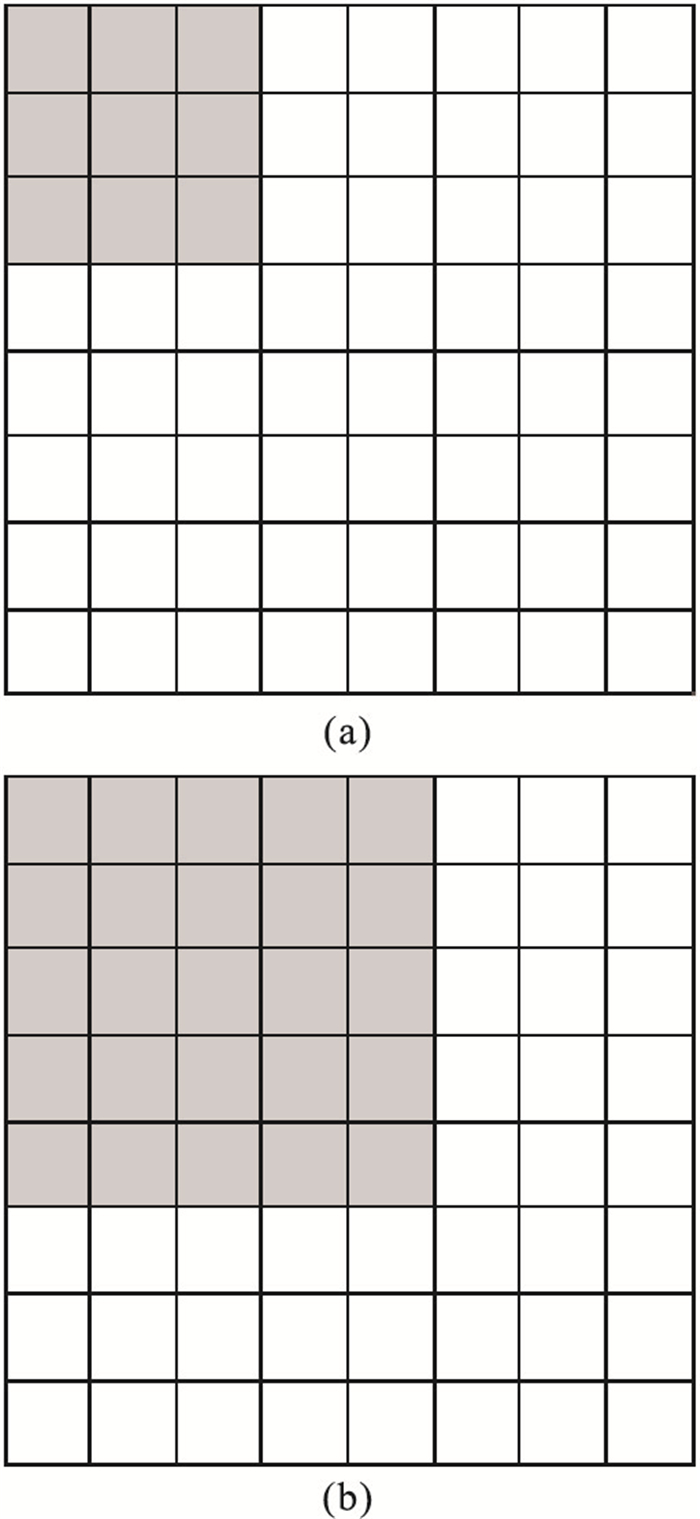图 2 经典卷积(a，卷积核大小为3×3)与扩充卷积(b，扩充因子为2，卷积核大小增至5×5)对比

2 模型测试

 $\sigma = l\sqrt {\frac{1}{{MN}}\sum\limits_{i = 1}^M {\sum\limits_{s = 1}^N {{{({\mathit{\boldsymbol{x}}_{t,s}} - u)}^2}} } }$ (7)

 ${\rm{PSNR}} = 10\lg \frac{{{\rm{max}}({\mathit{\boldsymbol{x}}^2})}}{{{\rm{MSE}}}}$ (8)
 ${\rm{SNR}} = 10{\rm{lg}}\frac{{\sum\limits_{t = 1}^M {\sum\limits_{s = 1}^N {{{({\mathit{\boldsymbol{x}}_{t,s}})}^2}} } }}{{\sum\limits_{t = 1}^N {\sum\limits_{s = 1}^N {{{({\mathit{\boldsymbol{x}}_{t,s}} - {{\mathit{\boldsymbol{\hat x}}}_{t,s}})}^2}} } }}$ (9)
 ${\rm{SSIM}}(\mathit{\boldsymbol{x}},\mathit{\boldsymbol{\hat x}}) = \frac{{(2{u_\mathit{\boldsymbol{x}}}{u_{\mathit{\boldsymbol{\hat x}}}} + {C_1})(2{\sigma _{\mathit{\boldsymbol{x\hat x}}}} + {C_2})}}{{({u_\mathit{\boldsymbol{x}}}^2 + {u_{\mathit{\boldsymbol{\hat x}}}}^2 + {C_1})({\sigma _\mathit{\boldsymbol{x}}}^2 + {\sigma _{\mathit{\boldsymbol{\hat x}}}}^2 + {C_2}){\rm{ }}}}$ (10)

2.1 网络层次结构分析

2.1.1 时频域联合损失函数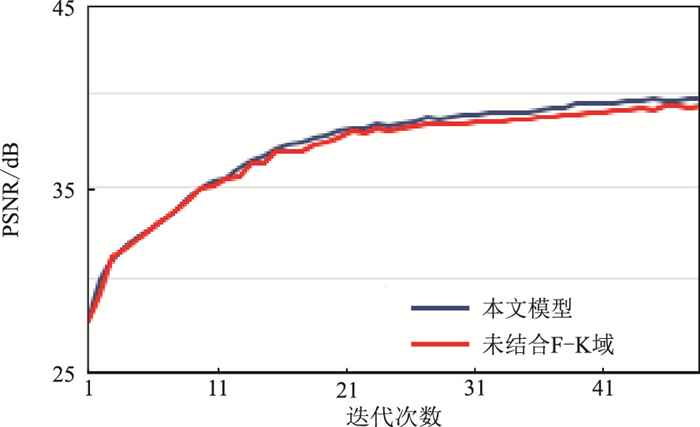图 3 不同模型计算误差函数的PSNR对比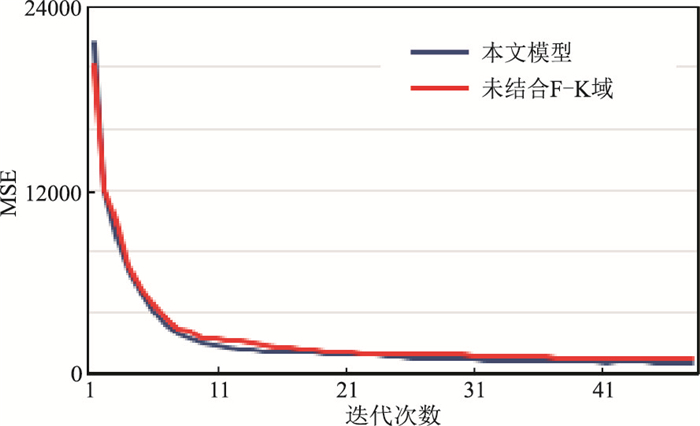图 4 不同模型计算误差函数的MSE对比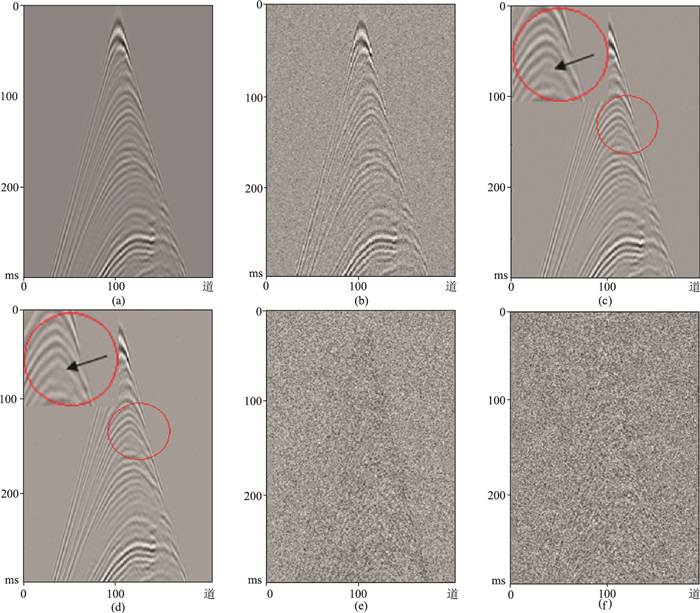图 5 不同模型去噪效果对比图 (a)原始地震数据；(b)加入l为0.05的高斯随机噪声后的地震数据；(c)未结合F-K域计算损失函数的去噪结果；(d)结合F-K域计算损失函数的去噪结果；(e)未结合F-K域计算损失函数的残差剖面；(f)结合F-K域计算损失函数的残差剖面图c和图d的左上方较大圆形区域为中间较小圆形区域的放大展示。图c中：PSNR=39.0700dB，SNR=33.1892dB，SSIM=0.8553。图d中：PSNR=39.6300dB，SNR=33.7171dB，SSIM=0.8920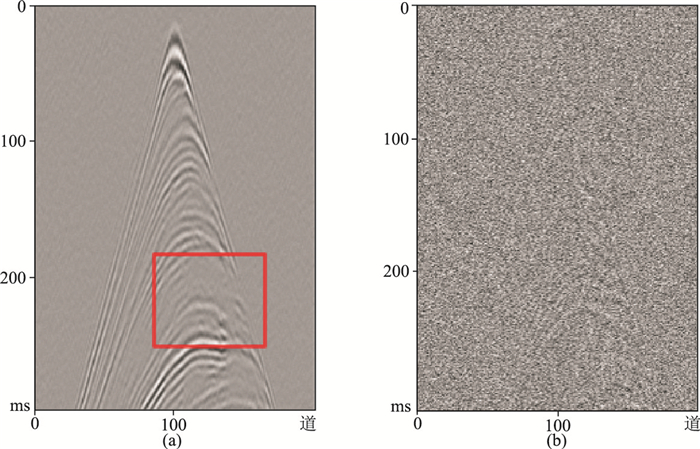图 6 仅含F-K域计算目标函数的去噪结果(a)和残差剖面(b) 图a中：PSNR=36.6800dB，SNR=29.3225dB，SSIM=0.8130
2.1.2 扩充卷积

l为0.03的条件下，训练过程中PSNR收敛情况如图 7a所示，策略A和策略B模型分别训练23和30次达到收敛状态，而策略C需要训练37次才能达到收敛状态。相比之下，前两者的训练时间效率更高。另外，通过对比三者PSNR，发现本文网络模型在训练集上最终收敛的PSNR为44dB，比策略A的PSNR约提高3dB。在测试集上，本文模型的平均PSNR为42.3dB，比策略A的PSNR平均约提高2.4dB。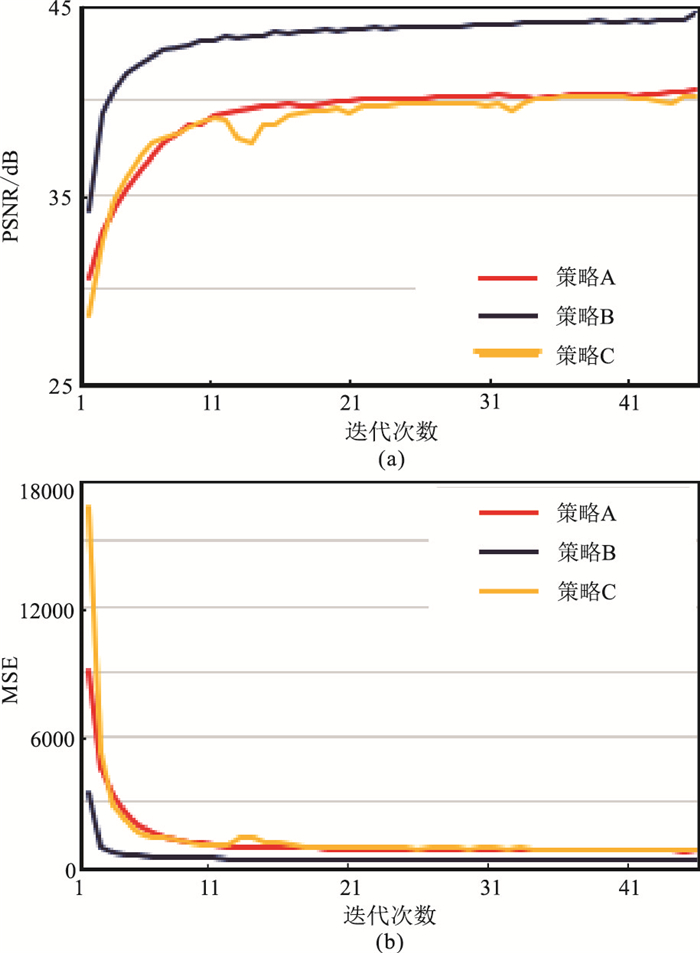图 7 不同网络结构模型训练PSNR (a)和MSE(b)对比

l为0.03的条件下，训练过程中MSE收敛情况如图 7b所示，可见相比于策略A和策略B，策略C的MSE曲线波动更剧烈且不稳定。相比于策略A，本文网络模型的MSE曲线在训练达到稳定时的误差更小，去噪效果更好。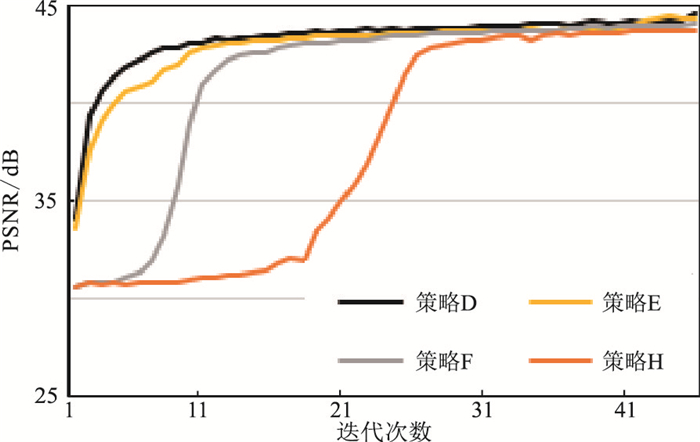图 8 各组扩充因子模型的收敛情况对比
2.1.3 残差学习策略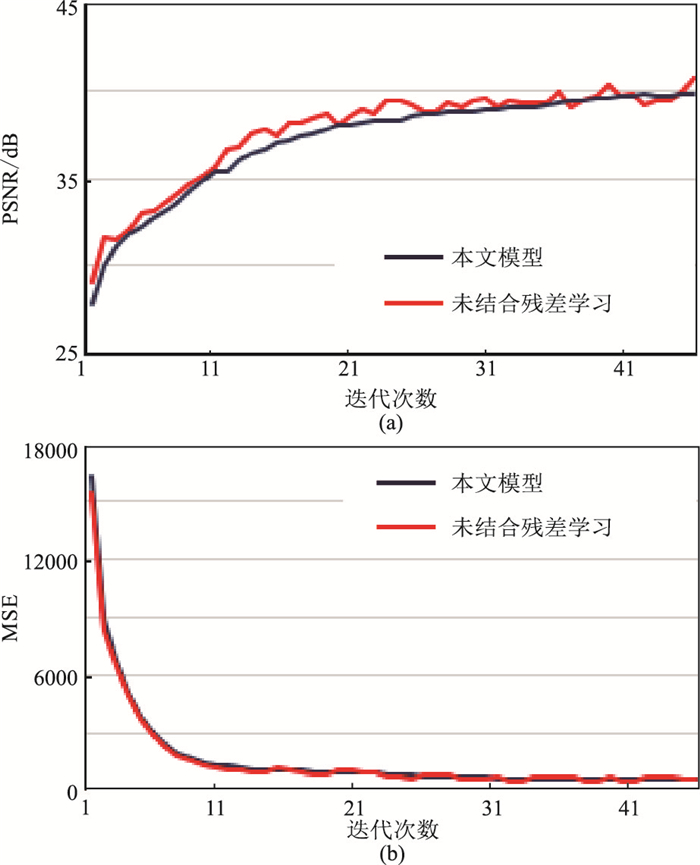图 9 有、无残差学习模型的PSNR(a)与MSE(b)对比

2.1.4 残差网络中加入BN层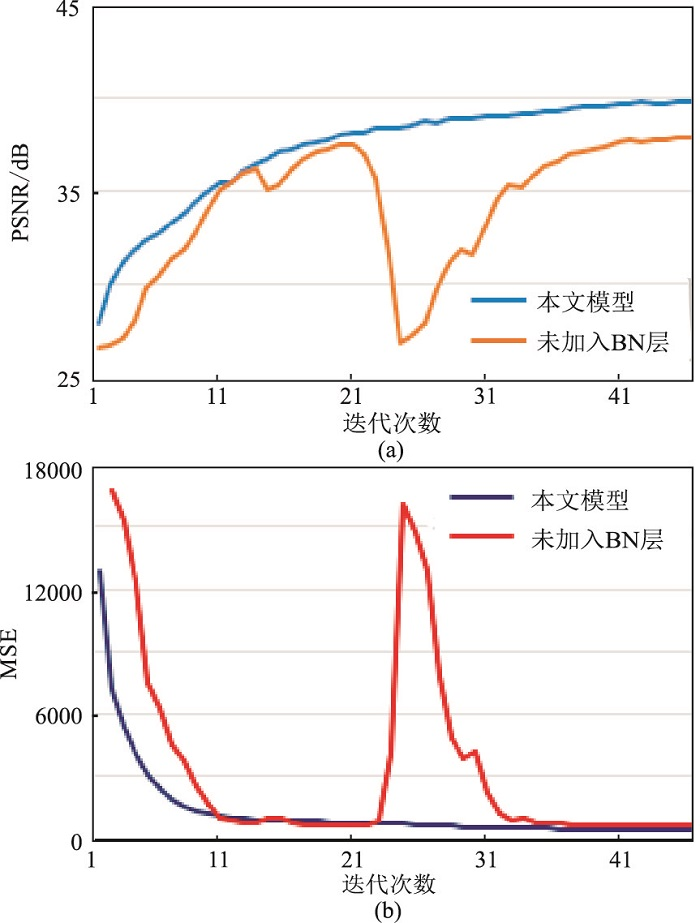图 10 有、无BN层模型的PSNR(a)与MSE(b)对比

2.1.5 网络模型的部分特征图分析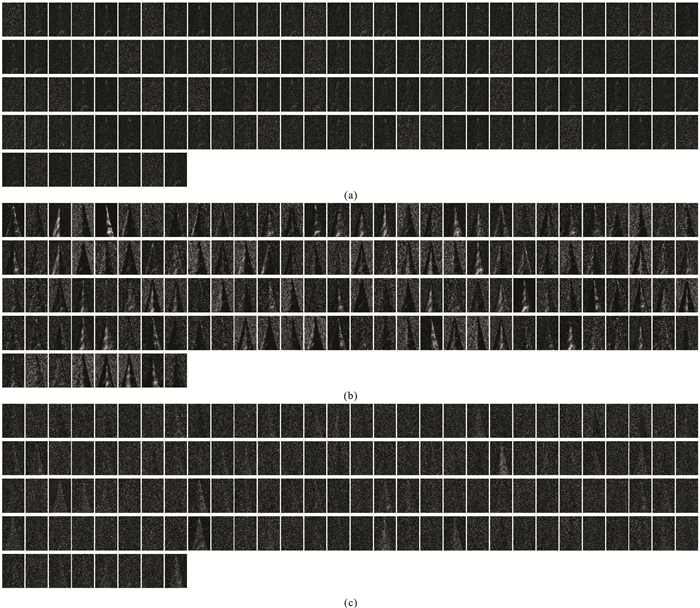图 11 网络模型去噪过程中经过不同层网络后的特征图 (a)第1层；(b)第4层；(c)第8层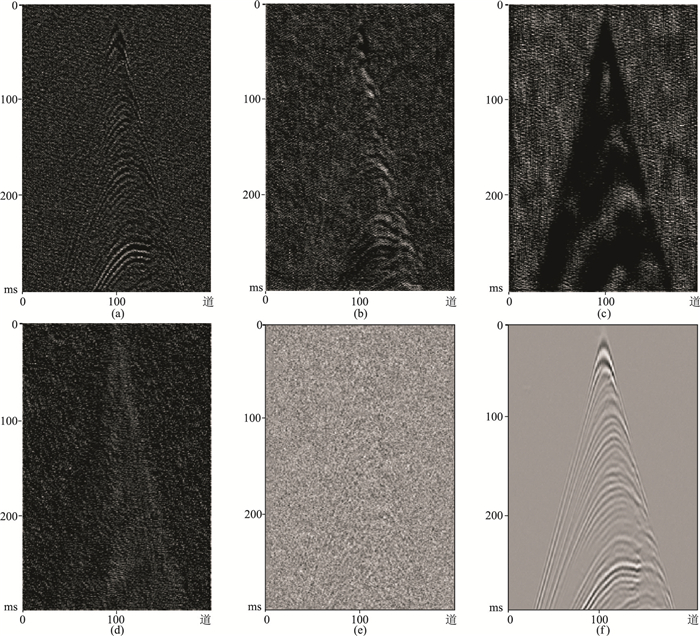图 12 部分特征图的放大展示 (a)第2层第1个卷积核提取的特征；(b)第4层第1个卷积核提取的特征；(c)第6层第1个卷积核提取的特征；(d)第8层第1个卷积核提取的特征；(e)经过第10层网络后得到的特征；(f)最终去噪结果
2.2 本文方法与其他同类方法对比

2.2.1 相同强度随机噪声情况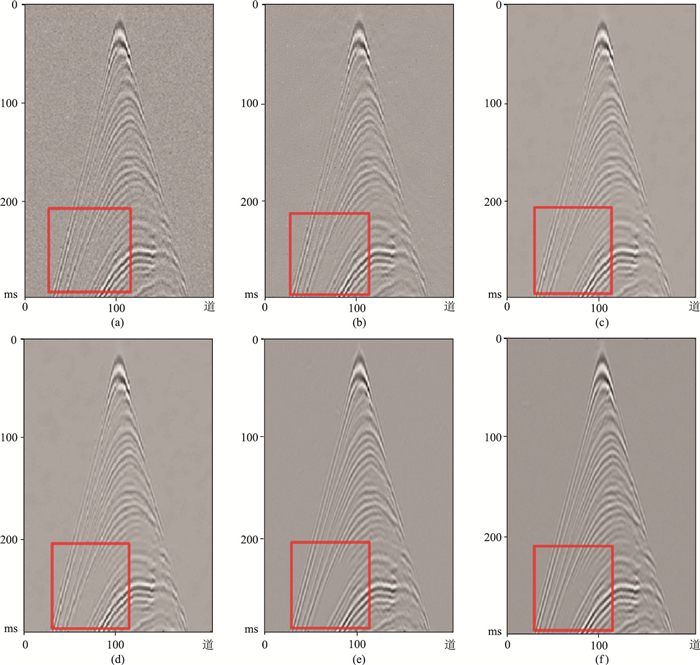图 13 不同去噪算法在相同强度高斯随机噪声下的去噪效果对比 (a)加入l为0.03的高斯随机噪声后地震数据；(b)Curvelet变换；(c)DCT超完备字典；(d)K-SVD自适应学习超完备字典；(e)DnCNN方法；(f)本文方法表 1 不同方法去噪效果对比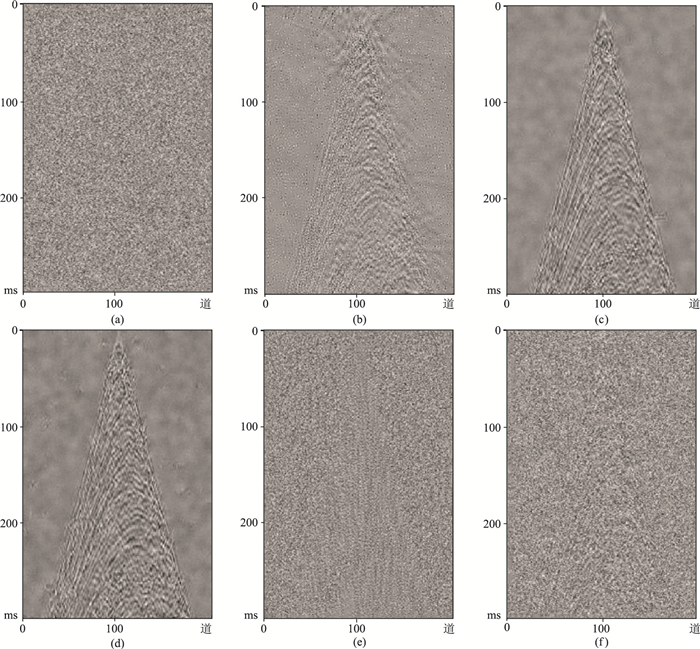图 14 不同去噪算法在相同强度高斯随机噪声下的残差剖面对比 (a)加入l为0.03的高斯随机噪声后；(b)Curvelet变换噪声压制后；(c)DCT超完备字典噪声压制后；(d)K-SVD自适应学习超完备字典噪声压制后；(e)DnCNN噪声压制后；(f)本文方法噪声压制后
2.2.2 不同强度随机噪声情况表 2 不同算法在加入较低强度高斯随机噪声下噪声压制后的SNR对比 dB表 3 不同算法在加入较高强度高斯随机噪声下噪声压制后的SNR对比 dB
2.3 本文模型对不同强度随机噪声的去噪效果对比表 4 不同强度噪声的训练数据对测试数据噪声压制后的SNR对比 dB

(1) 以原始数据加入l=0.03噪声等级的测试集为例(表 4l=0.03的列)，采用l=0.03强度的噪声训练的网络取得了该列中最高的SNR值，该列的其他训练噪声强度因子l=0.02、0.04、0.05的训练集得到的网络，去噪效果比较接近。

(2) 以l=0.03噪声等级的训练集为例(表 4l=0.03的行)，该模型不仅对l=0.03的噪声强度去噪有效，对l=0.02、0.04的噪声强度也表现出了较好的去噪效果。因此本文算法对于测试集噪声分布与训练集分布较为接近时具有一定的泛化能力。

3 实际资料处理 3.1 数据训练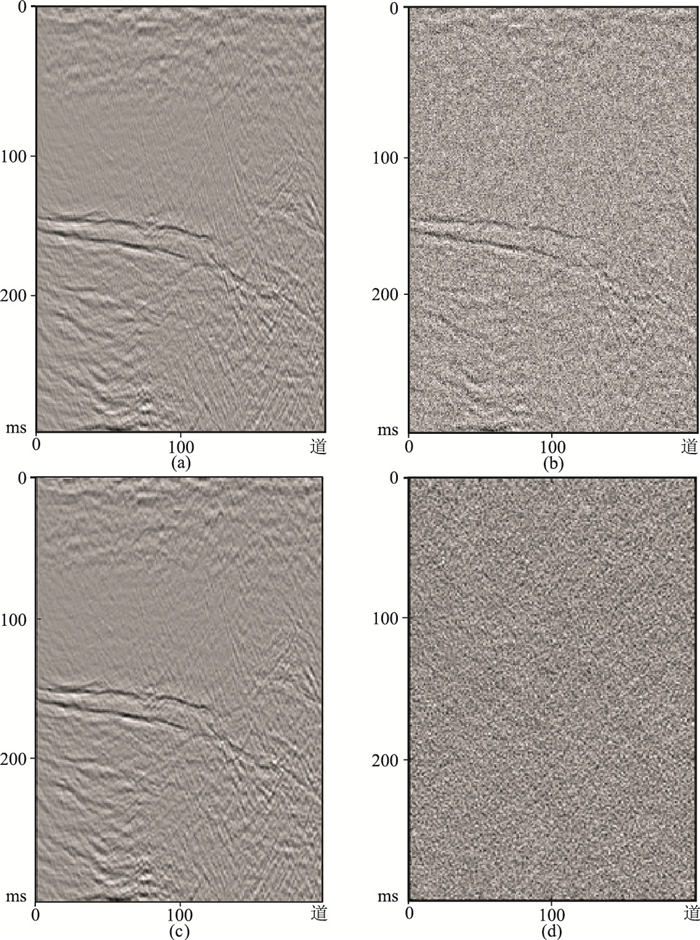图 15 任意样本及训练效果展示 (a)原始地震数据；(b)加入强度l为0.03的高斯随机噪声后数据；(c)第50次迭代训练的去噪结果；(d)第50次迭代训练去噪后的残差剖面。图b和图c的PSNR、SNR、SSIM分别为27.4771dB、23.2793dB、0.6185和41.85162dB、37.4036dB、0.9578

3.2 数据测试

3.2.1 经过预处理的实际数据实验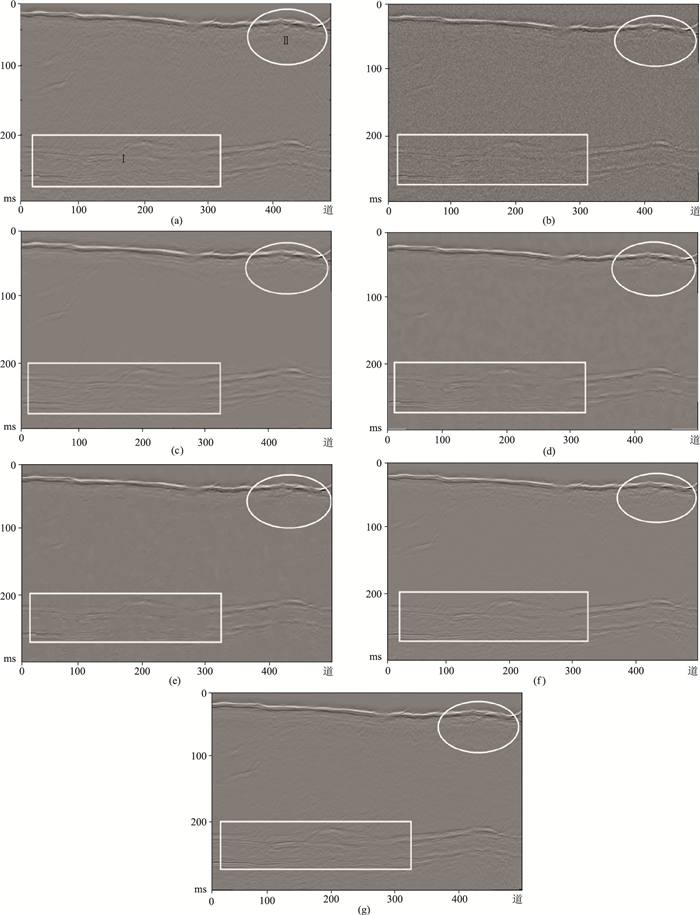图 16 不同方法对预处理后实际数据的噪声压制效果对比 (a)预处理后原始叠后海洋地震数据；(b)加入l为0.03的高斯随机噪声后数据；(c)Curvelet变换方法；(d)DCT超完备字典方法；(e)K-SVD自适应学习超完备字典方法；(f)DnCNN方法；(g)本文方法表 5 不同方法去噪后的PSNR、SNR、SSIM对比
3.2.2 原始实际数据实验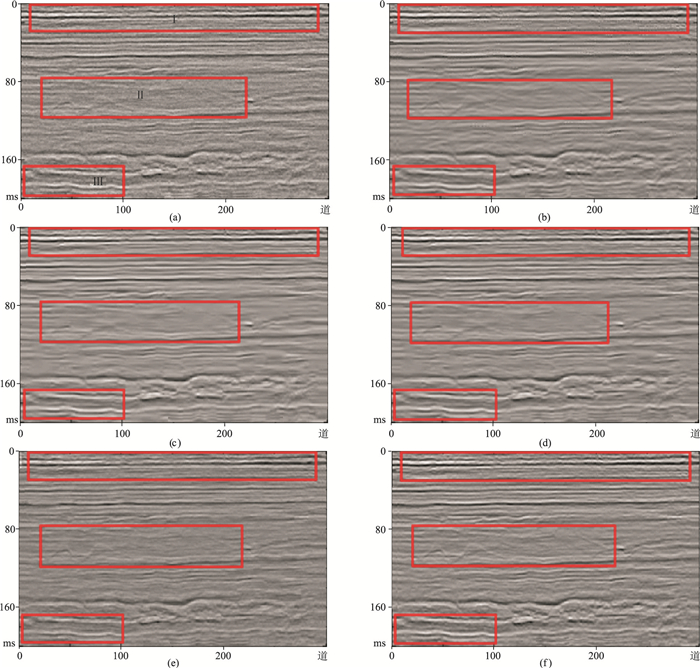图 17 原始实际数据不同方法噪声压制结果对比 (a)原始叠后地震数据；(b)Curvelet变换方法；(c)DCT超完备字典方法；(d)K-SVD自适应学习超完备字典方法；(e)DnCNN方法；(f)本文方法
4 结论

  张军华, 吕宁, 田连玉, 等. 地震资料去噪方法综合评述[J]. 石油地球物理勘探, 2005, 40(增刊1): 121-127. ZHANG Junhua, LYU Ning, TIAN Lianyu, et al. A comprehensive review of seismic data denoising me-thods[J]. Oil Geophysical Prospecting, 2005, 40(S1): 121-127.  胡天跃. 地震资料叠前去噪技术的现状与未来[J]. 地球物理学进展, 2002, 17(2): 218-223. HU Tianyue. The current situation and future of seismic data prestack noise attenuation techniques[J]. Progress in Geophysics, 2002, 17(2): 218-223. DOI:10.3969/j.issn.1004-2903.2002.02.005  关新平, 赵立兴, 唐英干. 图像去噪混合滤波方法[J]. 中国图象图形学报, 2005, 10(3): 332-337. GUAN Xinping, ZHAO Lixing, TANG Yinggan. Mixed filter for image denoising[J]. Journal of Image and Graphics, 2005, 10(3): 332-337. DOI:10.3969/j.issn.1006-8961.2005.03.013  王伟, 高静怀, 陈文超, 等. 基于结构自适应中值滤波器的随机噪声衰减方法[J]. 地球物理学报, 2012, 55(5): 1732-1741. WANG Wei, GAO Jinghuai, CHEN Wenchao, et al. Random seismic noise suppression via structure-adaptive median filter[J]. Chinese Journal of Geophysics, 2012, 55(5): 1732-1741.  Alsdorf D. Noise reduction in seismic data using Fourier correction coefficient filtering[J]. Geophysics, 1997, 62(5): 1617-1627. DOI:10.1190/1.1444264  Trad D, Ulrych T, Sacchi M. Latest view of sparse Radon transforms[J]. Geophysics, 2003, 68(10): 386-399.  Cao S, Chen X. The second-generation wavelet transform and its application in denoising of seismic data[J]. Applied Geophysics, 2005, 2(2): 70-74. DOI:10.1007/s11770-005-0034-4  章珂, 刘贵忠, 邹大文, 等. 二进小波变换方法的地震信号分时分频去噪处理[J]. 地球物理学报, 1996, 39(2): 265-271. ZHANG Ke, LIU Guizhong, ZOU Dawen, et al. Seismic data time-frequency domain denoising based on dyadic wavelet transform[J]. Chinese Journal of Geophysics, 1996, 39(2): 265-271. DOI:10.3321/j.issn:0001-5733.1996.02.013  高静怀, 毛剑, 满蔚仕, 等. 叠前地震资料噪声衰减的小波域方法研究[J]. 地球物理学报, 2006, 49(4): 1155-1163. GAO Jinghuai, MAO Jian, MAN Weishi, et al. On the denoising method of prestack seismic data in wavelet domain[J]. Chinese Journal of Geophysics, 2006, 49(4): 1155-1163. DOI:10.3321/j.issn:0001-5733.2006.04.030  Elad M, Aharon M. Image denoising via sparse and redundant representations over learned dictionaries[J]. IEEE Transactions on Image Processing, 2006, 15(12): 3736-3745. DOI:10.1109/TIP.2006.881969  Tang G, Ma J W, Yang H Z. Seismic data denoising based on learning-type overcomplete dictionaries[J]. Applied Geophysics, 2012, 9(1): 27-32. DOI:10.1007/s11770-012-0310-z  张岩, 任伟建, 唐国维. 应用结构聚类字典学习压制地震数据随机噪声[J]. 石油地球物理勘探, 2018, 53(6): 1119-1127. ZHANG Yan, REN Weijian, TANG Guowei. Random noise suppression on seismic data based on structured-clustering dictionary learning[J]. Oil Geophysical Prospecting, 2018, 53(6): 1119-1127.  张玉玺, 刘洋, 张浩然, 等. 基于深度学习的多属性盐丘自动识别方法[J]. 石油地球物理勘探, 2020, 55(3): 475-483. ZHANG Yuxi, LIU Yang, ZHANG Haoran, et al. Multiattribute automatic interpretation of salt domes based on deep learning[J]. Oil Geophysical Prospecting, 2020, 55(3): 475-483.  李海山, 陈德武, 吴杰, 等. 叠前随机噪声深度残差网络压制方法[J]. 石油地球物理勘探, 2020, 55(3): 493-503. LI Haishan, CHEN Dewu, WU Jie, et al. Pre-stack random noise suppression with deep residual network[J]. Oil Geophysical Prospecting, 2020, 55(3): 493-503.  王钰清, 陆文凯, 刘金林, 等. 基于数据增广和CNN的地震随机噪声压制[J]. 地球物理学报, 2019, 62(1): 421-433. WANG Yuqing, LU Wenkai, LIU Jinlin, et al. Random seismic noise attenuation based on data augmentation and CNN[J]. Chinese Journal of Geophysics, 2019, 62(1): 421-433.  Zhang K, Zuo W M, Chen Y J, et al. Beyond a Gaussian denoiser:Residual learning of deep CNN for image denoising[J]. IEEE Transactions on Image Processing, 2017, 26(7): 3142-3155. DOI:10.1109/TIP.2017.2662206  Yang L Q, Chen W, Liu W, et al. Random noise attenuation based on residual convolutional neural network in seismic datasets[J]. IEEE Access, 2020, 8: 30271-30286. DOI:10.1109/ACCESS.2020.2972464  曹仰杰, 贾丽丽, 陈永霞, 等. 生成式对抗网络及其计算机视觉应用研究综述[J]. 中国图象图形学报, 2018, 23(10): 1433-1449. CAO Yangjie, JIA Lili, CHEN Yongxia, et al. Review of computer vision based on generative adversarial networks[J]. Journal of Image and Graphics, 2018, 23(10): 1433-1449. DOI:10.11834/jig.180103  Zhang M, Liu Y, Chen Y K. Unsupervised seismic random noise attenuation based on deep convolutional neural network[J]. IEEE Access, 2019, 7: 179810-179822. DOI:10.1109/ACCESS.2019.2959238  Chen Y K, Zhang M, Bai M, et al. Improving the signal-to-noise ratio of seismological datasets by unsupervised machine learning[J]. Seismological Research Letters, 2019, 90(4): 1552-1564.  罗仁泽, 李阳阳. 一种基于RUnet卷积神经网络的地震资料随机噪声压制方法[J]. 石油物探, 2020, 59(1): 51-59. LUO Renze, LI Yangyang. Random seismic noise attenuation based on RUnet convolutional neural network[J]. Geophysical Prospecting for Petroleum, 2020, 59(1): 51-59.  韩卫雪, 周亚同, 池越. 基于深度学习卷积神经网络的地震数据随机噪声去除[J]. 石油物探, 2018, 57(6): 862-869. HAN Weixue, ZHOU Yatong, CHI Yue. Deep lear-ning convolutional neural networks for random noise attenuation in seismic data[J]. Geophysical Prospecting for Petroleum, 2018, 57(6): 862-869. DOI:10.3969/j.issn.1000-1441.2018.06.008  Yu S W, Ma J W, Wang W L. Deep learning for denoising[J]. Geophysics, 2019, 84(6): V333-V350. DOI:10.1190/geo2018-0668.1  Wang F, Chen S C. Residual learning of deep convolutional neural network for seismic random noise attenuation[J]. IEEE Geoscience and Remote Sensing, 2019, 16(8): 1314-1318. DOI:10.1109/LGRS.2019.2895702  Zhao Y X, Li Y, Dong X T, et al. Low-frequency noise suppression method based on improved DnCNN in desert seismic data[J]. IEEE Geoscience and Remote Sensing, 2019, 16(5): 811-815. DOI:10.1109/LGRS.2018.2882058  Dong X T, Li Y, Yang B J. Desert low-frequency noise suppression by using adaptive DnCNNs based on the determination of high-order statistic[J]. Geophysical Journal International, 2019, 219(2): 1281-1299. DOI:10.1093/gji/ggz363  Liu P J, Zhang H Z, Lian W, et al. Multilevel wavelet convolutional neural networks[J]. IEEE Access, 2019, 7: 74973-74985. DOI:10.1109/ACCESS.2019.2921451  Zhu W Q, Mousavi S M, Beroza G C. Seismic signal denoising and decomposition using deep neural networks[J]. IEEE Transactions on Geoscience and Remote Sensing, 2019, 57(11): 9476-9488. DOI:10.1109/TGRS.2019.2926772  李传朋, 秦品乐, 张晋京. 基于深度卷积神经网络的图像去噪研究[J]. 计算机工程, 2017, 43(3): 253-260. LI Chuanpeng, QIN Pinle, ZHANG Jinjing. Research on image denoising based on deep convolutional neural network[J]. Computer Engineering, 2017, 43(3): 253-260. DOI:10.3969/j.issn.1000-3428.2017.03.042